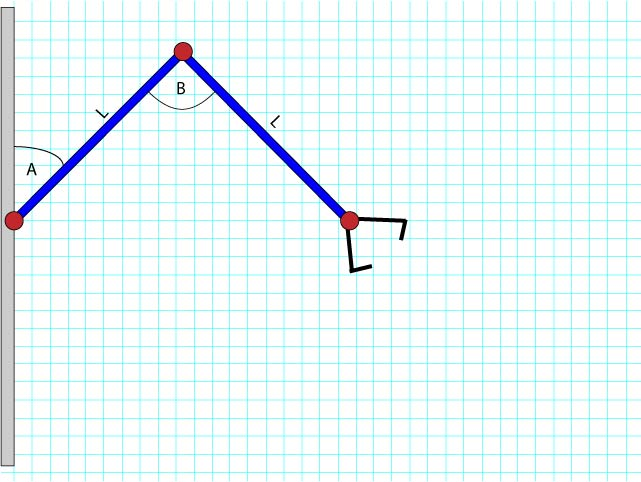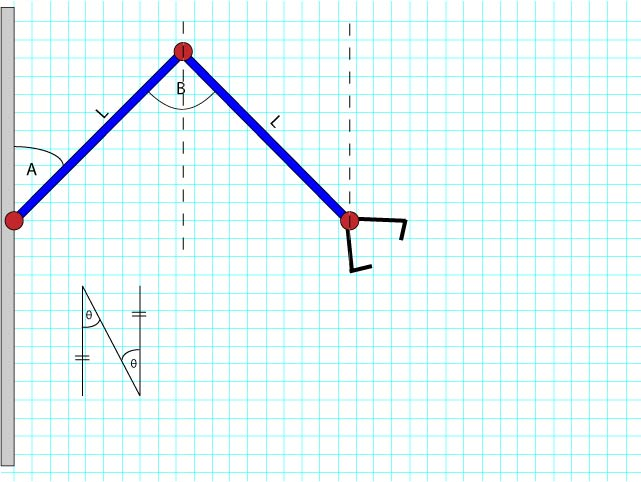A useful technique when trying to solve a problem is to see if there’s a simpler version of the problem that you can solve.

In this case, I figured it would be fairly trivial to make a robot arm that only moves backwards and forwards on the x-axis. As it turns out, all this requires, is for angle B to be exactly twice angle A.Make sense? How about now?That’s right; the lines I drew are parallel, so the alternate angles are equal. Double it for the entire triangle, Bob’s your uncle.

In the next entry, I’ll describe how we perform ranging (determine what angles to actually use based on the distance to the target).